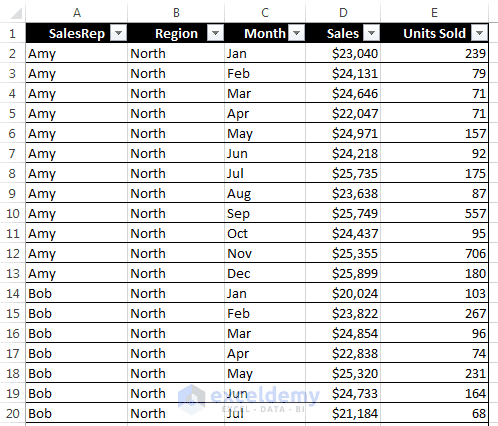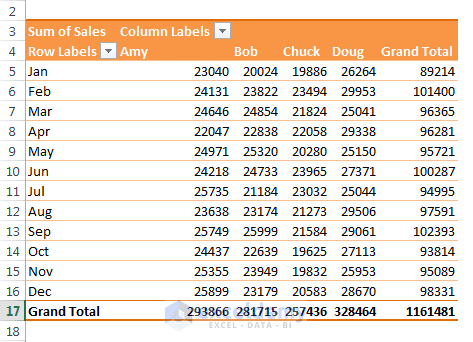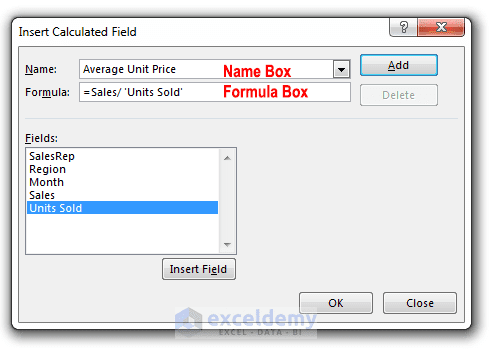Disclosure: This post may contain affiliate links, meaning when you click the links and make a purchase, we receive a commission.

# How to Create Pivot Table Calculated Field for Average

In this article, we shall show how you can create a pivot table calculated field for showing the average of two existing data fields.

Calculated fields and calculated items are two confusing aspects of pivot tables. Due to complexity, many pivot table users avoid these two features. But these features are really useful, and they aren’t complicated if you understand well how they work.

You can’t use the standard Excel formulas to create calculated fields and calculated items. Actually, you will not enter the formulas into cells. Rather, you will enter formulas to create these features in a dialog box. The formulas you have entered into the dialog box and the pivot table data will be stored.

We shall make you understand these features using some examples. We have used a sample file and our examples are based on this sample file. The following figure shows part of a table in that file.This figure shows part of a table. We shall use this table to work with calculated fields and calculated items.

The table shown in the above figure consists of five columns and 48 rows. Each row of the table contains monthly sales amount for a particular sales representative. For example, Bob is a sales representative for the North region, he sold 267 units in the month of February and his total sales were \$23,882.

The following figure shows a pivot table created from the table. To create this pivot table, we have placed Month field in the Rows area, SalesRep field in the Columns area and Sales field in the Values area.This pivot table is created from the above table. We have placed Month, SalesRep in Rows and Columns area, and Sales in Values area.

## Creating Pivot Table Calculated Field Average

A pivot table is a special type of range. You can’t insert new rows or columns within the pivot table. In summary, we can say that you can’t insert formulas to perform calculations with the data in a pivot table. However, you can create calculated fields for a pivot table. A calculated field consists of a calculation that involves other fields.

With the help of a calculated field (added in a pivot table), you can display new information. A calculated field is an alternative to creating a new column field in your source data. In most cases, you will find that it is easier to create a new column field in your source data with a formula that performs the expected calculation. But when your data is from an external source and you can’t manipulate the data source, you can use calculated field feature.

See our above figure and observe the pivot table. The boss of the company may want to know the average unit price sold by Bob in the month of February. To find out the average unit price, divide the Sales field by the Units Sold field. We are going to add a new field to the pivot table that will show the average unit price.

Read More: Data appropriate for a pivot table

We have used the following procedure to create a calculated field that shows the average unit price data:

### Step 1:

Select any cell within the pivot table.

### Step 2:

Choose PivotTable Tools ➪ Analyze ➪ Calculations ➪ Fields, Items & Sets ➪ Calculated Field. The Insert Calculated Field dialog box will appear.

### Step 3:

There are two fields in the Insert Calculated Field dialog box. One is Name Box and another is Formula Box. Enter a descriptive name in the Name Box and input the formula in the Formula Box. The formula can use any worksheet functions and use any fields from the data source. In our example, we have entered Average Unit Price in the Name box, and this formula in the Formula box: =Sales/’Units Sold’.This figure shows the Insert Calculated Field dialog box. Enter a descriptive name in the name box and specify a formula in the formula box.

### Step 4:

Click Add button in the dialog box to add this new field in the Fields section.

### Step 5:

Click OK to close the Insert Calculated Field dialog box.

Note: You can enter the formula manually by typing it in the formula box or by double-clicking items in the Fields list box. When you double-click an item, the item will be transferred to the formula box. Because the Units Sold field contains a space, Excel adds single quotes around this field name when it is entered in the formula box.

Read More: How to Insert a Calculated Item into Excel Pivot Table!

We are almost done. After you have created the calculated field (Average Unit Price), Excel automatically adds this field as a new column in the pivot table with its calculated values. The calculated field also appears in the PivotTable Fields task pane. You can treat this new field just like any other field, but you can’t move it to the Rows, Columns, or Filters areas like other fields. This field must remain in the Values area.

The following figure shows the pivot table after we have added the calculated field Average Unit Price. Some labels have been shortened to extend readability. To shorten a label, select the label, press F2 and change the label. I have applied some styling for better visualization.

Tip: The formulas that you develop can also use worksheet functions, but the functions can’t refer to cells or named ranges.